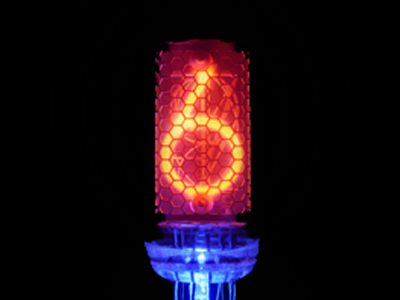6 is the digit in the hundredths' place in 17.2685.

# Place Value 2 (Medium)

Welcome to the second of our Medium level Eleven-Plus maths quizzes on Place Value. Like the first, this quiz gives you the chance to test your knowledge of place values in four-digit decimal numbers.

Unlike in whole numbers, the values are worked out by reading from right to left. But ONLY digits AFTER the decimal point are counted.

Here are the place values for decimal numbers:
• First number after decimal point – Tenths
• Second number after decimal point – Hundredths
• Third number after decimal point – Thousandths
• Fourth number after decimal point – Ten thousandths

There are no Ones after a decimal point – remember this and you should manage to answer all the questions in this quiz correctly. Let’s see if you can – good luck!

1.
What is the place value of 2 in 0.3279?
The tenths' place
The hundredths' place
The thousandths' place
The ten thousandths' place
Reading from left to right immediately after the decimal point: 2 is in the hundredths' place
2.
What is the digit in the ten thousandths' place in 92.8163?
8
1
6
3
Reading from left to right immediately after the decimal point: 3 is in the ten thousandths' place
3.
What is the digit in the tenths' place in 903.7068?
7
0
6
8
Reading from left to right immediately after the decimal point: 7 is in the tenths' place
4.
What is the digit in the thousandths' place in 76,256.3901?
6
9
0
1
Reading from left to right immediately after the decimal point: 0 is in the thousandths' place
5.
What is the place value of 4 in 28.0749?
The tenths' place
The hundredths' place
The thousandths' place
The ten thousandths' place
Reading from left to right immediately after the decimal point: 4 is in the thousandths' place
6.
What is the place value of 9 in 207.6913?
The tenths' place
The hundredths' place
The thousandths' place
The ten thousandths' place
Reading from left to right immediately after the decimal point: 9 is in the hundredths' place
7.
What is the place value of 9 in 28,456.0179?
The ten thousandths' place
The thousandths' place
The hundredths' place
The tenths' place
Reading from left to right immediately after the decimal point: 9 is in the ten thousandths' place
8.
What is the digit in the tenths' place in 17.8219?
8
2
1
9
Reading from left to right immediately after the decimal point: 8 is in the tenths' place
9.
What is the digit in the hundredths' place in 926.0583?
0
5
8
3
Reading from left to right immediately after the decimal point: 5 is in the hundredths' place
10.
What is the place value of 5 in 107.2956?
The tenths' place
The hundredths' place
The thousandths' place
The ten thousandths' place
Reading from left to right immediately after the decimal point: 5 is in the thousandths' place
Author:  Frank Evans